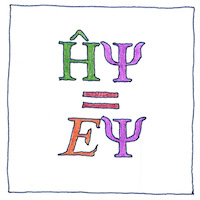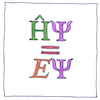# 1926 Schrödinger equation

## The book of science

Tom Sharp

 Erwin Schrödinger quantum mechanics## Schrödinger equation

• If a thing could be a wave or a particle
• then a classical trajectory cannot describe it.
• Erwin Schrödinger derived an equation
• for how a wave function changes over time.

## The operation of time is unitary

• Time changes each thing independently.
• See how quickly the system
• processes each quantum of energy,
• its potential, its mass, and the momentums
• of all its possible paths.
• Multiple things in a system affect each other.
• This could be a contraction,
• or time always operates on the system,
• or we have stopped thinking of mere time.
• Time operates on the system because multiple particles
• acting together have only one wave function.

## Multiples

• Multiple interpretations,
• multiple solutions,
• and multiple implications
• of which multiple universes
• and multiple multiplications
• are possible
• and possibly
• probable.

I have illustrated the time-independent Schrödinger equation because it is simpler. The time-dependent Schrödinger equation is more explicit about space and time:

$\mathit{i}\mathit{ħ}\frac{\delta }{\delta \mathit{t}}\Psi \left(\mathbf{r},t\right)=\mathit{Ĥ}\Psi \left(\mathbf{r},t\right)$

See also in The book of science: